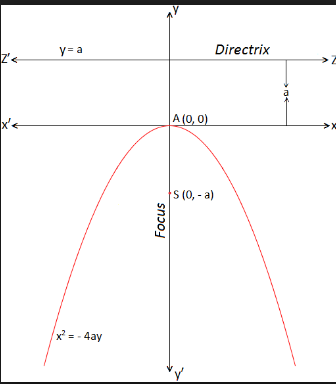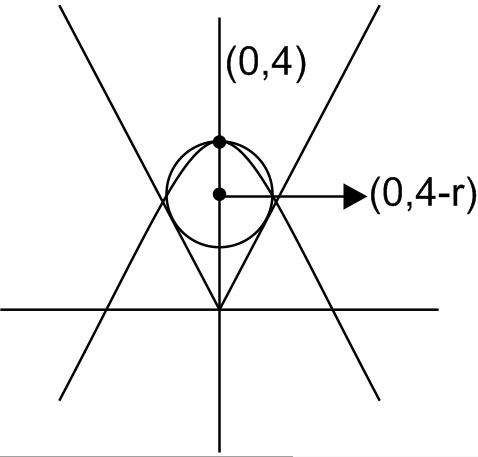Filters

Q&A - Ask Doubts and Get Answers
Q

Help me answer: - Co-ordinate geometry - JEE Main

The radius of a circle, having minimum area, which touches the curve y=4−x2

and the lines,is:

• Option 1)• Option 2)• Option 3)• Option 4)103 Views

As we learnt in

Standard equation of parabola -

- whereinand concept

Perpendicular distance of a point from a line -

- wherein

is the distance from the line .This figure shows the circle with least area satisfying the conditions.

Circle touches y=x;

Option 1)Incorrect

Option 2)Correct

Option 3)Incorrect

Option 4)Incorrect

Exams
Articles
Questions